Hostname: page-component-cd4964975-8tfrx Total loading time: 0 Render date: 2023-03-29T11:16:16.233Z Has data issue: true Feature Flags: { "useRatesEcommerce": false } hasContentIssue true

# INTERPRETING A FIELD IN ITS HEISENBERG GROUP

Published online by Cambridge University Press:  23 December 2021

## Abstract

We improve on and generalize a 1960 result of Maltsev. For a field F, we denote by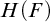$H(F)$ the Heisenberg group with entries in F. Maltsev showed that there is a copy of F defined in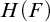$H(F)$ , using existential formulas with an arbitrary non-commuting pair of elements as parameters. We show that F is interpreted in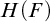$H(F)$ using computable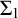$\Sigma _1$ formulas with no parameters. We give two proofs. The first is an existence proof, relying on a result of Harrison-Trainor, Melnikov, R. Miller, and Montalbán. This proof allows the possibility that the elements of F are represented by tuples in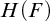$H(F)$ of no fixed arity. The second proof is direct, giving explicit finitary existential formulas that define the interpretation, with elements of F represented by triples in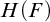$H(F)$ . Looking at what was used to arrive at this parameter-free interpretation of F in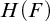$H(F)$ , we give general conditions sufficient to eliminate parameters from interpretations.

## MSC classification

Type
Article
Information
The Journal of Symbolic Logic , September 2022 , pp. 1215 - 1230
© The Author(s), 2021. Published by Cambridge University Press on behalf of The Association for Symbolic Logic

## Access options

Get access to the full version of this content by using one of the access options below. (Log in options will check for institutional or personal access. Content may require purchase if you do not have access.)

## References

Calvert, W., Cummins, D. F., Knight, J. F., and Miller, S., Comparing classes of finite structures . Algebra and Logic, vol. 43 (2004), pp. 374392.CrossRefGoogle Scholar
Friedman, H. and Stanley, L., A Borel reducibility theory for classes of countable structures, this Journal, vol. 54 (1989), pp. 894–914.Google Scholar
Harrison-Trainor, M., Melnikov, A., Miller, R., and Montalbán, A., Computable functors and effective interpretability, this Journal, vol. 82 (2017), pp. 77–97.Google Scholar
Harrison-Trainor, M., Miller, R., and Montalbán, A., Borel functors and infinitary interpretations, this Journal, vol. 83 (2018), pp. 1434–1456.Google Scholar
Hirschfeldt, D. R., Khoussainov, B., Shore, R. A., and Slinko, A. M., Degree spectra and computable dimensions in algebraic structures . Annals of Pure and Applied Logic, vol. 115 (2002), pp. 71113.CrossRefGoogle Scholar
Kalimullin, I., Algorithmic reducibilities of algebraic structures . Journal of Logic and Computation, vol. 22 (2012), pp. 831843.CrossRefGoogle Scholar
Maltsev, A., Some correspondences between rings and groups . Matematicheskii Sbornik, New Series, vol. 50 (1960), pp. 257266 (in Russian).Google Scholar
Mekler, A. H., Stability of nilpotent groups of class 2 and prime exponent, this Journal, vol. 46 (1981), pp. 781–788.Google Scholar
Miller, R., Poonen, B., Schoutens, H., and Shlapentokh, A., A computable functor from graphs to fields, this Journal, vol. 83 (2018), pp. 326–348.Google Scholar
Montalbán, A., Computable Structure Theory: Within the Arithmetic, Perspectives in Logic, Cambridge University Press, Cambridge, 2021.CrossRefGoogle Scholar
Scott, D., Logic with denumerably long formulas and finite strings of quantifiers , The Theory of Models (Addison, J. W., Henkin, L., and Tarski, A., editors), North-Holland, Amsterdam, 1965, pp. 329341.Google Scholar